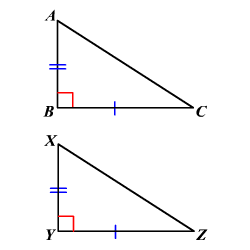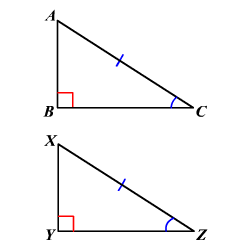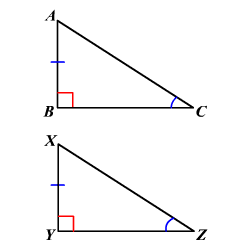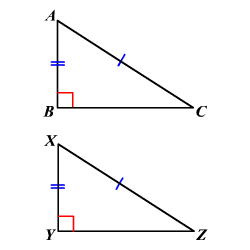# Right Triangle Congruence

### Leg-Leg Congruence

If the legs of a right triangle are congruent to the corresponding legs of another right triangle, then the triangles are congruent.In the figure, $\stackrel{¯}{AB}\cong \stackrel{¯}{XY}\text{\hspace{0.17em}}\text{\hspace{0.17em}}\text{and}\text{\hspace{0.17em}}\text{\hspace{0.17em}}\stackrel{¯}{BC}\cong \stackrel{¯}{YZ}$ .

So, $\Delta ABC\cong \Delta XYZ$ .

### Hypotenuse-Angle Congruence

If the hypotenuse and an acute angle of a right triangle are congruent to the hypotenuse and corresponding acute angle of another right triangle, then the triangles are congruent.In the figure, $\stackrel{¯}{AC}\cong \stackrel{¯}{XZ}\text{\hspace{0.17em}}\text{\hspace{0.17em}}\text{and}\text{\hspace{0.17em}}\text{\hspace{0.17em}}\angle C\cong \angle Z$ .

So, $\Delta ABC\cong \Delta XYZ$ .

### Leg-Angle Congruence

If one leg and an acute angle of a right triangle are congruent to one leg and the corresponding acute angle of another right triangle, then the triangles are congruent.In the figure, $\stackrel{¯}{AB}\cong \stackrel{¯}{XY}\text{\hspace{0.17em}}\text{\hspace{0.17em}}\text{and}\text{\hspace{0.17em}}\text{\hspace{0.17em}}\angle C\cong \angle Z$ .

So, $\Delta ABC\cong \Delta XYZ$ .

### Hypotenuse-Leg Congruence

If the hypotenuse and a leg of a right triangle are congruent to the hypotenuse and corresponding leg of another right triangle, then the triangles are congruent.In the figure, $\stackrel{¯}{AB}\cong \stackrel{¯}{XY}\text{\hspace{0.17em}}\text{\hspace{0.17em}}\text{and}\text{\hspace{0.17em}}\text{\hspace{0.17em}}\stackrel{¯}{AC}\cong \stackrel{¯}{XZ}$ .

So, $\Delta ABC\cong \Delta XYZ$ .